# Symmetry Worksheet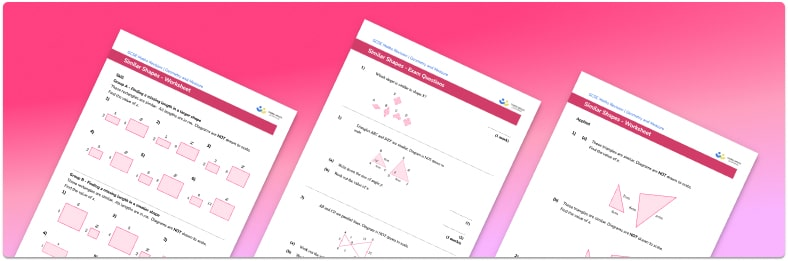• This field is for validation purposes and should be left unchanged.

You can unsubscribe at any time (each email we send will contain an easy way to unsubscribe). To find out more about how we use your data, see our privacy policy.

• Section 1 of the symmetry worksheet contains 36 skills-based symmetry questions, in 3 groups to support differentiation
• Section 2 contains 4 applied symmetry questions with a mix of worded problems and deeper problem solving questions
• Section 3 contains 4 foundation and higher level GCSE exam style symmetry questions
• Answers and a mark scheme for all questions are provided
• Questions follow variation theory with plenty of opportunities for students to work independently at their own level
• All questions created by fully qualified expert secondary maths teachers
• Suitable for GCSE maths revision for AQA, OCR and Edexcel exam boards

### Symmetry at a glance

Symmetry is the existence of identical parts within a shape, either next to each other or around an axis. There are two main types of symmetry; line symmetry and rotational symmetry.

In line symmetry, which is sometimes called reflective symmetry, the identical parts are a reflection of each other. We identify lines that we can draw so that one side of the line is a reflection of the other. These are called lines of symmetry, or mirror lines, since each side is a mirror image of the other. Shapes often have multiple lines of symmetry and so to identify the number of lines of symmetry that a shape has, we need to rotate our mirror line around and draw lines of symmetry that we notice.

In rotational symmetry the identical parts are around an axis. The order of rotational symmetry of a shape tells us how many times a shape will fit back on top of itself exactly as it is rotated around an axis.

There are many different symmetrical shapes. Some have lines of symmetry only, some have rotational symmetry only and some have both. For regular polygons such as equilateral triangles, squares and regular hexagons, the number of lines of symmetry is equal to the order of rotational symmetry and both are equal to the number of sides of the polygon.

We can apply our knowledge of symmetry to other areas of maths such as graphs. Graphs of some functions have line or rotational symmetry.

Looking forward, students can then progress to additional geometry worksheets, for example a 3D shapes worksheet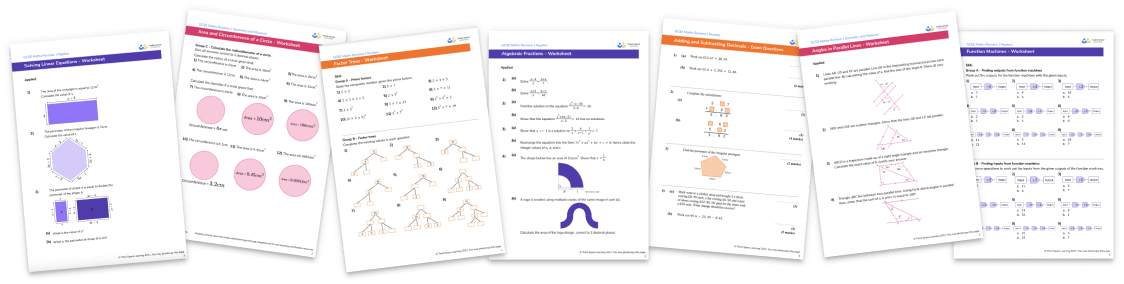For more teaching and learning support on Geometry our GCSE maths lessons provide step by step support for all GCSE maths concepts.

## Related worksheets

Plans And Elevations Worksheet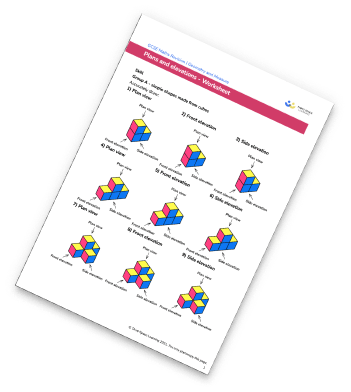Perimeter Worksheet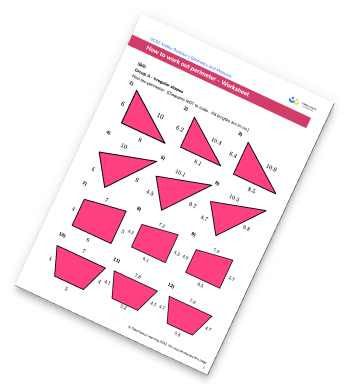Surface Area Worksheets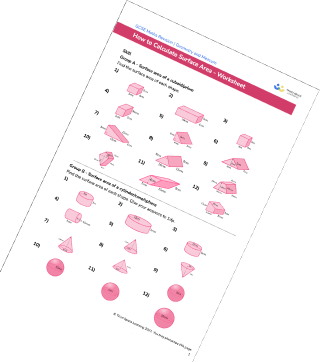Volume Worksheets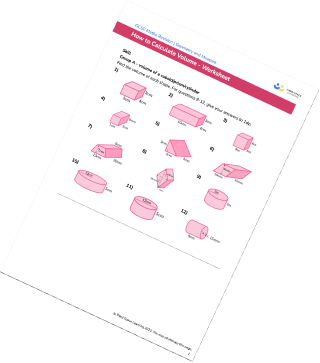## Do you have KS4 students who need more focused attention to succeed at GCSE?There will be students in your class who require individual attention to help them succeed in their maths GCSEs. In a class of 30, it’s not always easy to provide.

Help your students feel confident with exam-style questions and the strategies they’ll need to answer them correctly with our dedicated GCSE maths revision programme.

Lessons are selected to provide support where each student needs it most, and specially-trained GCSE maths tutors adapt the pitch and pace of each lesson. This ensures a personalised revision programme that raises grades and boosts confidence.

Find out more